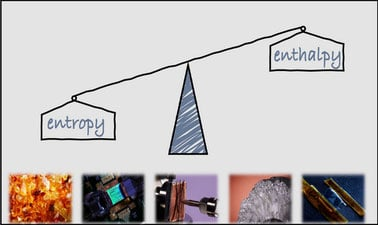# Thermodynamics of Materials

Thermodynamics of Materials describes the fundamental competition between energy and disorder that determines the state of materials at equilibrium.## There is one session available:

After a course session ends, it will be archived.
Estimated 16 weeks
9–12 hours per week
Self-paced

This course describes the fundamental competition between energy and disorder that determines the state of materials at equilibrium. It introduces the laws of thermodynamics, the concept of equilibrium, and thermodynamic potentials. Both classical and statistical interpretations of entropy are presented, but the emphasis of the course is on classical thermodynamics. Topics covered include unary systems, reacting chemical systems, and binary phase diagrams. Emphasis is placed on the concept of constrained equilibrium, the mathematical structure of classical thermodynamics, and on free energy-composition diagrams that underpin binary phase diagrams. Computational thermodynamics is also covered, and thermodynamics software is used throughout the course. The course also emphasizes the uses of thermodynamics is materials selection and process design, and the importance of materials data.

### At a glance

• Institution: MITx
• Subject: Engineering
• Level: Intermediate
• Prerequisites:

Single and multivariable calculus; partial differential equations; data analysis; general chemistry

• Language: English

# What you'll learn

Skip What you'll learn
• The laws of thermodynamics, how to define a thermodynamic system, how to identify dependent and independent thermodynamics variables, and how to predict equilibrium states.
• How to construct unary and binary phase diagrams from thermodynamic principles and data, and how to use phase diagrams to predict process outcomes.
• How to predict the equilibrium state of reacting systems.
• How to find and assess thermodynamic data, and how to use it.
• How to understand and use computational thermodynamics software works.
• The microscopic, molecular origins of enthalpy and entropy.

# Syllabus

Skip Syllabus

Section A: Equilibrium

• Lecture 1 - Introduction to thermodynamics: Enthalpy, entropy, and an atomic view
• Lecture 2 - Systems, states, and material properties
• Lecture 3 - Processes and the First Law
• Lecture 4 - Irreversible processes, the Second Law, and equilibrium
• Lecture 5 - The combined statement and differential forms
• Lecture 6 - Equilibrium conditions

Section B: Phase Diagrams

• Lecture 7-8 - Unary systems and phase diagrams
• Lecture 9 - The three dees of thermodynamics
• Lectures 10-11 - Systems of reacting gases
• Lecture 12 - Introduction to binary phase diagrams
• Lecture 13 - The lever rule
• Lecture 14-15 - Partial molar properties and the common tangent construction
• Lecture 16 - Heterogeneous binary systems and ideal solutions
• Lectures 17-18 - Non-ideal solutions: Dilute and regular models
• Lecture 19 - Heterogeneous binary systems: Gibbs phase rule; eutectic, and peritectic reactions
• Lecture 20-21 - Reference states
• Lecture 22 - Intermediate phases and line compounds
• Lecture 23 - Ternary phase diagrams
• Lecture 24 - Reacting systems: Metal oxidation

Section C: Foundations

• Lecture 25 - Clausius’ statement and the Carnot efficiency limit
• Lecture 26 - Reversible & irreversible heat engines
• Lecture 27 - Introduction to statistical thermodynamics

# Learner testimonials

Skip Learner testimonials

“I loved how planned his lectures were, how good he was at hinting at more complex topics occasionally to spark interest, and how he would expressly tell us to look at things in the book or online.”

“I ended up really liking this class!”

“Thermo is tough, but you managed to show me how to extract some joy from it.”